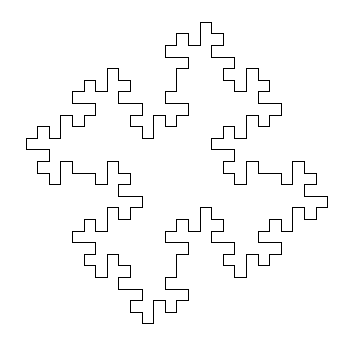Want to share your content on R-bloggers? click here if you have a blog, or here if you don't.

Don’t worry about a thing ’cause every little thing gonna be alright (Three Little Birds, Bob Marley)

One of my favourite books is The Computational Beauty of Nature by Gary William Flake where there is a fantastic chapter about fractals in which I discovered the L-Systems.

L-Systems were conceived  in 1968 by Aristide Lindenmayer, a Hungarian biologist, as a mathematical description of plant growth. Apart from the Wikipedia, there are many places on the Internet where you can read about them. If you are interested, don’t miss The Algorithmic Beauty of Plants, an awesome book by Przemysław Prusinkiewicz that you can obtain here for free.

Roughly speaking, a L-System is a very efficient way to make drawings. In its simplest way consists in two different actions: draw a straigh line and change the angle. This is just what you need, for example, to draw a square: draw a straigh line of  any length, turn 90 degrees (without drawing), draw another straigh line of the same length, turn 90 degrees in the same direction, draw, turn and draw again. Denoting `F` as the action of drawing a line of length d and `+` as turning 90 degrees right, the whole process to draw a square can be represented as `F+F+F+F`.

L-Systems are quite simple to program in R. You only need to substitute the rules iteratively into the axiom (I use `gsubfn` function to do it) and split the resulting chain into parts with `str_extract_all`, for example. The result is a set of very simple actions (draw or turn) that can be visualized with `ggplot` and its path geometry. There are four important parameters in L-Systems:

• The seed of the drawing, called axiom
• The substitutions to be applied iteratively, called rules
• How many times to apply substitutions, called depth
• Angle of each turning

For example, let’s define the next L-System:

• Axiom: `F-F-F-F`
• Rule: `F → F−F+F+FF−F−F+F`

The rule means that every `F` must be replaced by `F−F+F+FF−F−F+F` while `+` means right turning and `-` left one. After one iteration, the axiom is replaced by `F-F+F+FF-F-F+F-F-F+F+FF-F-F+F-F-F+F+FF-F-F+F-F-F+F+FF-F-F+F` and iterating again, the new string is `F-F+F+FF-F-F+F-F-F+F+FF-F-F+F+F-F+F+FF-F-F+F+F-F+F+FF-F-F+FF-F+F+FF-F-F+F-F-F+F+FF-F-F+F-F-F+F+FF-F-F+F+F-F+F+FF-F-F+F-F-F+F+FF-F-F+F-F-F+F+FF-F-F+F+F-F+F+FF-F-F+F+F-F+F+FF-F-F+FF-F+F+FF-F-F+F-F-F+F+FF-F-F+F-F-F+F+FF-F-F+F+F-F+F+FF-F-F+F-F-F+F+FF-F-F+F-F-F+F+FF-F-F+F+F-F+F+FF-F-F+F+F-F+F+FF-F-F+FF-F+F+FF-F-F+F-F-F+F+FF-F-F+F-F-F+F+FF-F-F+F+F-F+F+FF-F-F+F-F-F+F+FF-F-F+F-F-F+F+FF-F-F+F+F-F+F+FF-F-F+F+F-F+F+FF-F-F+FF-F+F+FF-F-F+F-F-F+F+FF-F-F+F-F-F+F+FF-F-F+F+F-F+F+FF-F-F+F`. As you can see, the length of the string grows exponentially. Converting last string into actions, produces this drawing, called Koch Island:It is funny how different axioms and rules produce very different drawings. I have done a Shiny App to play with L-systems. Although it is quite simple, it has two interesting features I would like to undeline:

• Delay reactions with `eventReactive` to allow to set depth and angle values before refreshing the plot
• Build a dynamic UI that reacts to user input depending on the curve choosen

There are twelve curves in the application: Koch Island (and 6 variations), cuadratic snowflake, Sierpinsky triangle, hexagonal Gosper, quadratic Gosper and Dragon curve. These are their plots:

The definition of all these curves (axiom and rules) can be found in the first chapter of the Prusinkiewicz’s book. The magic comes when you modify angles and colors. These are some examples among the infinite number of possibilities that can be created:

I enjoyed a lot doing and playing with the app. You can try it here. If you do a nice drawing, please let me know in Twitter or dropping me an email. This is the code of the App:

`ui.R`:

```library(shiny)

shinyUI(fluidPage(
titlePanel("Curves based on L-systems"),

sidebarLayout(
sidebarPanel(
selectInput("cur", "Choose a curve:",
c("","Koch Island",
"Koch Variation 1",
"Koch Variation 2",
"Koch Variation 3",
"Koch Variation 4",
"Koch Variation 5",
"Koch Variation 6",
"Sierpinsky Triangle",
"Dragon Curve",
"Hexagonal Gosper Curve",
selected = ""),

conditionalPanel(
condition = "input.cur != \"\"",
uiOutput("Iterations")),

conditionalPanel(
condition = "input.cur != \"\"",
uiOutput("Angle")),

conditionalPanel(
condition = "input.cur != \"\"",
selectInput("lic", label = "Line color:", choices = colors(), selected = "black")),

conditionalPanel(
condition = "input.cur != \"\"",
selectInput("bac", label = "Background color:", choices = colors(), selected = "white")),

conditionalPanel(
condition = "input.cur != \"\"",
actionButton(inputId = "go", label = "Go!",
style="color: #fff; background-color: #337ab7; border-color: #2e6da4"))

),
mainPanel(plotOutput("curve", height="550px", width = "100%"))
)

))
```

`server.R`:

```library(shiny)
library(gsubfn)
library(stringr)
library(dplyr)
library(ggplot2)
library(rlist)

shinyServer(function(input, output) {

curves=list(
list(name="Koch Island",
axiom="F-F-F-F",
rules=list("F"="F-F+F+FF-F-F+F"),
angle=90,
n=2,
alfa0=90),
axiom="-F",
rules=list("F"="F+F-F-F+F"),
angle=90,
n=4,
alfa0=90),
list(name="Koch Variation 1",
axiom="F-F-F-F",
rules=list("F"="FF-F-F-F-F-F+F"),
angle=90,
n=3,
alfa0=90),
list(name="Koch Variation 2",
axiom="F-F-F-F",
rules=list("F"="FF-F-F-F-FF"),
angle=90,
n=4,
alfa0=90),
list(name="Koch Variation 3",
axiom="F-F-F-F",
rules=list("F"="FF-F+F-F-FF"),
angle=90,
n=3,
alfa0=90),
list(name="Koch Variation 4",
axiom="F-F-F-F",
rules=list("F"="FF-F--F-F"),
angle=90,
n=4,
alfa0=90),
list(name="Koch Variation 5",
axiom="F-F-F-F",
rules=list("F"="F-FF--F-F"),
angle=90,
n=5,
alfa0=90),
list(name="Koch Variation 6",
axiom="F-F-F-F",
rules=list("F"="F-F+F-F-F"),
angle=90,
n=4,
alfa0=90),
list(name="Sierpinsky Triangle",
axiom="R",
rules=list("L"="R+L+R", "R"="L-R-L"),
angle=60,
n=6,
alfa0=0),
list(name="Dragon Curve",
axiom="L",
rules=list("L"="L+R+", "R"="-L-R"),
angle=90,
n=10,
alfa0=90),
list(name="Hexagonal Gosper Curve",
axiom="L",
rules=list("L"="L+R++R-L--LL-R+", "R"="-L+RR++R+L--L-R"),
angle=60,
n=4,
alfa0=60),
axiom="-R",
rules=list("L"="LL-R-R+L+L-R-RL+R+LLR-L+R+LL+R-LR-R-L+L+RR-",
"R"="+LL-R-R+L+LR+L-RR-L-R+LRR-L-RL+L+R-R-L+L+RR"),
angle=90,
n=2,
alfa0=90))

output\$Iterations <- renderUI({ if (input\$cur!="") curve=list.filter(curves, name==input\$cur) else curve=list.filter(curves, name=="Koch Island") iterations=list.select(curve, n) %>% unlist
numericInput("ite", "Depth:", iterations, min = 1, max = (iterations+2))
})

output\$Angle <- renderUI({ curve=list.filter(curves, name==input\$cur) angle=list.select(curve, angle) %>% unlist
numericInput("ang", "Angle:", angle, min = 0, max = 360)
})

data <- eventReactive(input\$go, { curve=list.filter(curves, name==input\$cur) axiom=list.select(curve, axiom) %>% unlist
rules=list.select(curve, rules)[]\$rules
alfa0=list.select(curve, alfa0) %>% unlist

for (i in 1:input\$ite) axiom=gsubfn(".", rules, axiom)
actions=str_extract_all(axiom, "\\d*\\+|\\d*\\-|F|L|R|\\[|\\]|\\|") %>% unlist

points=data.frame(x=0, y=0, alfa=alfa0)
for (i in 1:length(actions))
{
if (actions[i]=="F"|actions[i]=="L"|actions[i]=="R")
{
x=points[nrow(points), "x"]+cos(points[nrow(points), "alfa"]*(pi/180))
y=points[nrow(points), "y"]+sin(points[nrow(points), "alfa"]*(pi/180))
alfa=points[nrow(points), "alfa"]
points %>% rbind(data.frame(x=x, y=y, alfa=alfa)) -> points
}
else{
alfa=points[nrow(points), "alfa"]
points[nrow(points), "alfa"]=eval(parse(text=paste0("alfa",actions[i], input\$ang)))
}
}
return(points)
})

output\$curve <- renderPlot({
ggplot(data(), aes(x, y)) +
geom_path(color=input\$lic) +
coord_fixed(ratio = 1) +
theme(legend.position="none",
panel.background = element_rect(fill=input\$bac),
panel.grid=element_blank(),
axis.ticks=element_blank(),
axis.title=element_blank(),
axis.text=element_blank())
})

})
```